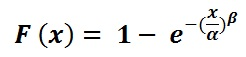## Weibull Distribution Calculator

 Alpha (α) Beta (β) X1 X2
 Result: P( X1 < X < X2 ) Mean Mode Median Variance Standard Deviation

The Weibull Distribution Calculator to calculate risk predictions with extremely small samples using a simple and useful graphical plot and precise failure analysis.

The calculator uses the input values of slopeα , β to generate the respective output values of mean, median, mode, standard deviation and variance.

### Weibull Distribution FormulaFor example, when Alpha (α) = 2, Beta (β) = 23, X1 = 2, X2 = 4, Then P( X1 < X < X2 ) = 0.0223, Mean = 16.2635, Mode = 20.3873, Median = 19.1488, Variance = 113.3571, Standard Deviation = 10.6469.

Thinkcalculator.com provides you helpful and handy calculator resources.